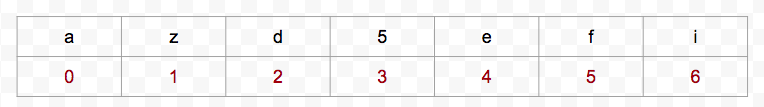# Time and Space Complexity

In the previous lessons you got introduced to different types of data structures; ordered collection, unordered collection, mutable and immutable structures etc.. But have you ever wondered what are the advantages and/or disadvantages of one structure over other in your application?

An algorithm's efficiency is measured by how less of the following resources of the computer it consumes:

• CPU (time) usage - refers to the Time Complexity
• RAM memory usage - refers to the Space Complexity

Although there could be other resources like network bandwidth etc., that may be of interest, but typically in computer science we are focused on time and space complexity

However, let us note the subtle difference between the real performance and complexity measurements;

• Performance: how much of real time or memory is actually used when a program is run. This depends on the machine configuration as well as the code
• Complexity: how much time and memory is required when the algorithm has to scale for larger inputs - represented as a function of input and hence does not depend on the specific machine.

In this lesson you will learn the time and space complexities of the algorithms that are used behind the scenes in these data structures.

#### Time Complexity

The time complexity of an algorithm refers to the amount of CPU time taken by an algorithm to run. The time required by the algorithm is computed by adding all the time required to run the "basic operations" that it performs; this includes arithmetic operations, assignment operation, comparison operations, read/write operations etc..

Let us now take a simple example of searching for an element in the various data structures that you were exposed to thus far, starting with the list

### Analyzing Time Complexity of a ListIn the above list if you want to find a particular element of interest is present or not, you have to iterate the list from the first index position all the way till you find the element. Once you find the element you stop the iteration. The number of iterations you need depends upon at what index position the element is present. Here is the simple algorithm to do that:

``````

def is_element_present(c, x):
for element in x:
if c == element:
return True
return False

list_1 = [ 'a', 'z', 'd', 5, 'e', 'f', 'i' ]
is_element_present('f', list_1)
``````

In the above algorithm;

• The best case scenario is 1 iteration; if the element that you are looking for is in the 0th index
• The worst case scenarios is 'N' iterations if the element that you are looking for is the last element of a list with 'N' elements

Inside the iteration, CPU time is used to compare two elements; but in other complex algorithms it may be doing more than just a comparison. So when we measure the complexity of the algorithm, we are not interested in the exact number of operations that are being performed. Instead, we are interested in the relation (or the function) of the number of operations to the problem size (N). In computer science we represent this worst case scenario with a Big O (a.k.a Big O) notation. For the list search that we just performed, the Big O representation of the element search is

O(N)

Which basically means that the worst case scenario of searching for an element in a list can be represented as a linear function of 'N'; means if the list is small, you find your element faster and if the list is super large, it takes a longer time and the exact time is proportional to the list size.

The above search time complexity is true for Tuple and also a String. O(N) is the time complexity of search for all sequences. What about a Set or Dictionary? Let us find out

### Analyzing Time Complexity of a Dictionary

Dictionary is not a sequence. You retrieve a value by looking up the 'key' and internally it uses a hash function to compute the hashcode and then jumps to the key location using the hashcode and retrieves the value. So a key look up takes the amount of time required to compute the hash value and hence it is in constant time and it does not matter how large the dictionary is, you can jump to your key in a constant time.

Hence the key look up time complexity of a dictionary is

O(1)

which basically means that the worst case scenario of key look up is in constant time – independent of the number of items.

This is true for a Set also since both Set and Dictionary are unordered collection and is internally built using the hash function.

While we only covered the key look up or element search in the above two examples; what about key insertion, key deletion, item insertion and deletion in sequences etc.. Each of these are computed in a similar way and each of these operations are represented with a Big O notation.

While in this short lesson, you learnt on time complexity, you can also compute the space complexity by taking into account how much RAM memory is consumed by an algorithm. However that is an exercise for you to research and explore.